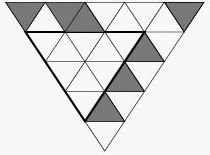## Saturday, August 28, 2010

### poj_1471_Triangles.cpp

Zoj1245, Poj1471, UVa00585,

## Problem:

Triangles
 Time Limit: 1000MS Memory Limit: 10000K Total Submissions: 1472 Accepted: 530
Description
It is always very nice to have little brothers or sisters. You can tease them, lock them in the bathroom or put red hot chili in their sandwiches. But there is also a time when all meanness comes back!

As you know, in one month it is Christmas and this year you are honored to make the big star that will be stuck on the top of the Christmas tree. But when you get the triangle-patterned silver paper you realize that there are many holes in it. Your little sister has already cut out smaller triangles for the normal Christmas stars. Your only chance is to find an algorithm that tells you for each piece of silver paper the size of the largest remaining triangle.

Given a triangle structure with white and black fields inside you must find the largest triangle area of white fields, as shown in the following figure.Input
The input contains several triangle descriptions. The first line of each description contains an integer n (1 <= n <= 100), which gives the height of the triangle. The next n lines contain characters of the set {space, #, -} representing the rows of the triangle, where `#' is a black and `-' a white field. The spaces are used only to keep the triangle shape in the input by padding at the left end of the lines. (Compare with the sample input. The first test case corresponds to the figure.)
For each triangle, the number of the characters `#' and `-' per line is odd and decreases from 2n - 1 down to 1.

The input is terminated by a description starting with n = 0.
Output
For each triangle in the input, first output the number of the triangle, as shown in the sample output. Then print the line "The largest triangle area is a.", where a is the number of fields inside the largest triangle that consists only of white fields. Note that the largest triangle can have its point at the top, as in the second case of the sample input.

Output a blank line after each test case.
Sample Input
```5
#-##----#
-----#-
---#-
-#-
-
4
#-#-#--
#---#
##-
-
0```
Sample Output
```Triangle #1
The largest triangle area is 9.

Triangle #2
The largest triangle area is 4.
```
Source
Southwestern European Regional Contest 1997

## Solution:

Dynamic programming.
f[i,j]=min(f[i-1,j-1],f[i-1,j+1])+1(map[i-1,j]=’-');
g[i,j]=min(g[i+1,j-1],g[i+1,j+1])+1(map[i+1,j]=’-');

## Source Code:

//Fri Jun 18 23:56:59 CDT 2010
#include <vector>
#include <list>
#include <map>
#include <set>
#include <deque>
#include <queue>
#include <stack>
#include <bitset>
#include <algorithm>
#include <functional>
#include <numeric>
#include <utility>
#include <sstream>
#include <iostream>
#include <iomanip>
#include <cstdio>
#include <cmath>
#include <cstdlib>
#include <cctype>
#include <string>
#include <cstring>
#include <cstdio>
#include <cmath>
#include <cstdlib>
#include <ctime>

using namespace std;

int main(int argc, char* argv[])
{
//  freopen("input.in", "r", stdin);
//  freopen("output.out", "w", stdout);
int N;
int ncase = 1;
while (cin >> N && N)
{
vector<string> v(N, string(2 * N - 1, 'b'));
vector<vector<int> > count1(N, vector<int> (2 * N - 1, 0));
vector<vector<int> > count2(N, vector<int> (2 * N - 1, 0));
for (int i = 0; i < N; i++)
{
for (int j = i; j < 2 * N - 1 - i; j++)
{
cin >> v[i][j];
}
}
int mmax = 0;
for (int i = 0; i < N; i++)
{
for (int j = i; j < 2 * N - 1 - i; j += 2)
{
if (v[i][j] == '-')
count1[i][j] = 1;
if (i - 1 >= 0)
{
if (v[i - 1][j] == '-' && v[i - 1][j - 1] == '-'
&& v[i - 1][j + 1] == '-')
{
count1[i][j] += min(count1[i - 1][j - 1],
count1[i - 1][j + 1]);
}
}
mmax = max(count1[i][j], mmax);
}
}
for (int i = N - 2; i >= 0; i--)
{
for (int j = i + 1; j < 2 * N - 1 - i; j += 2)
{
if (v[i][j] == '-')
count2[i][j] = 1;
if (i + 1 < N && v[i + 1][j - 1] == '-' && v[i + 1][j] == '-'
&& v[i + 1][j + 1] == '-')
count2[i][j] += min(count2[i + 1][j - 1], count2[i + 1][j
+ 1]);
mmax = max(mmax, count2[i][j]);
}
}
cout << "Triangle #" << ncase++ << endl;
cout << "The largest triangle area is " << mmax * mmax << "." << endl;
cout << endl;
}
//  fclose(stdin);
//  fclose(stdout);
return 0;
}